Question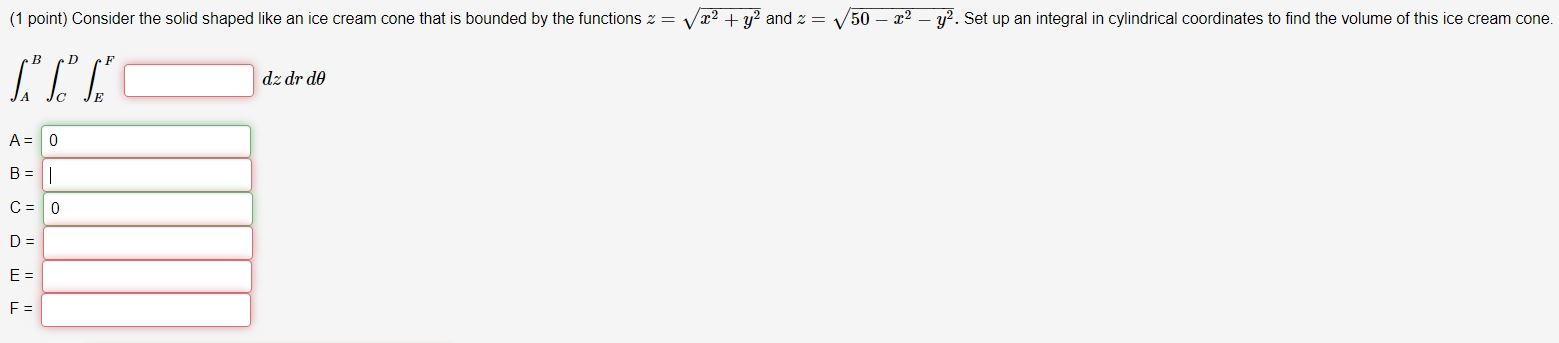Can anyone help me figure out the limits and what the function would be? Thank you!

We will convert into polar coordinates.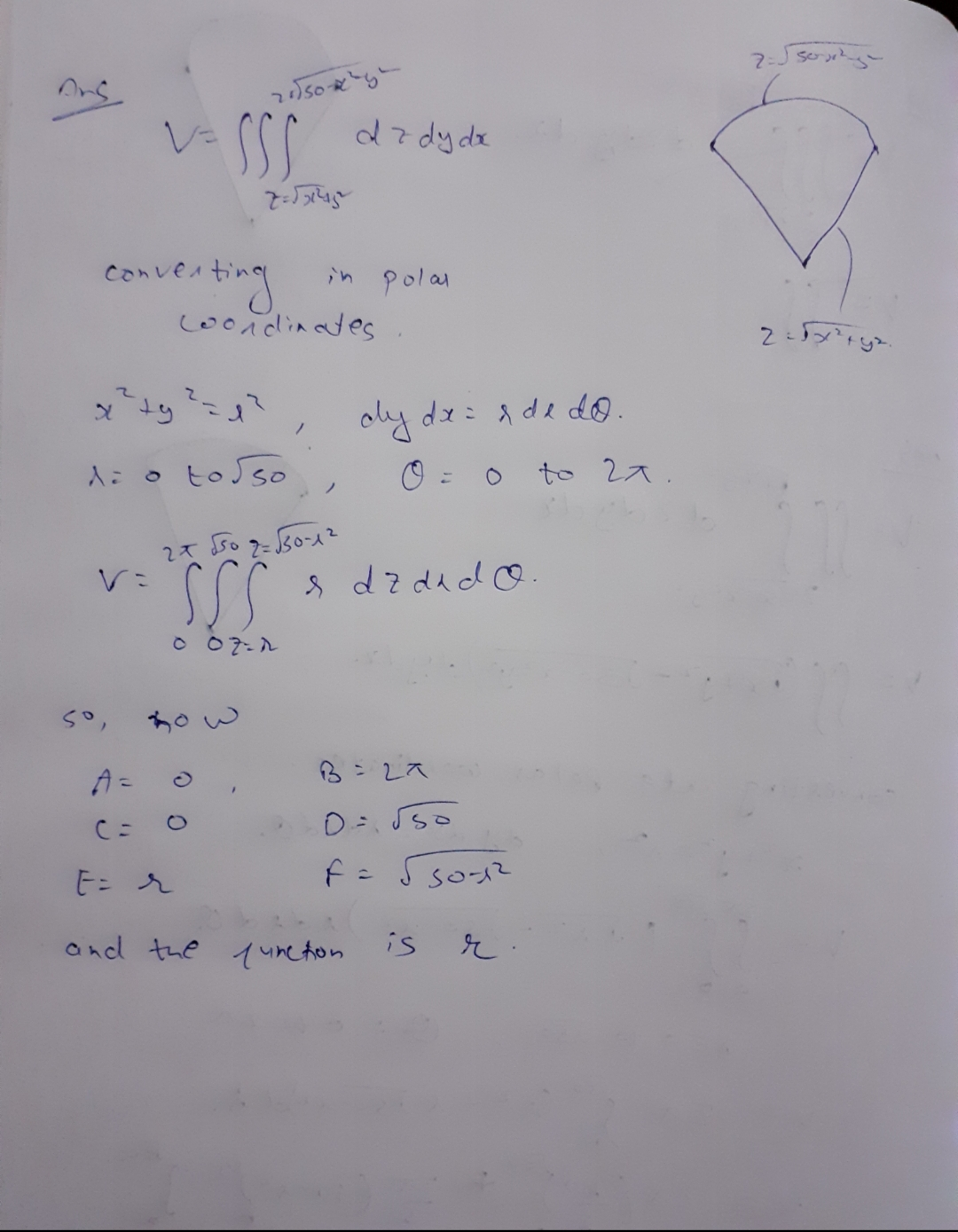#### Earn Coins

Coins can be redeemed for fabulous gifts.

Similar Homework Help Questions
• ### 7. (5 pts) By completing the limits and integrand, set up (without evaluating) an iterated inte-...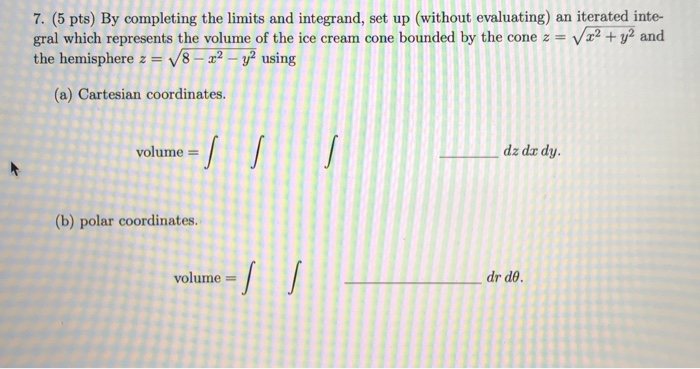7. (5 pts) By completing the limits and integrand, set up (without evaluating) an iterated inte- gral which represents the volume of the ice cream cone bounded by the cone z = V x2 + y2 and the hemisphere z = V8 - 22 - y2 using (a) Cartesian coordinates. volume = dz dar dy. (b) polar coordinates. volume = dr de.

• ### 7. (5 pts) By completing the limits and integrand, set up (without evaluating) an iterated inte-...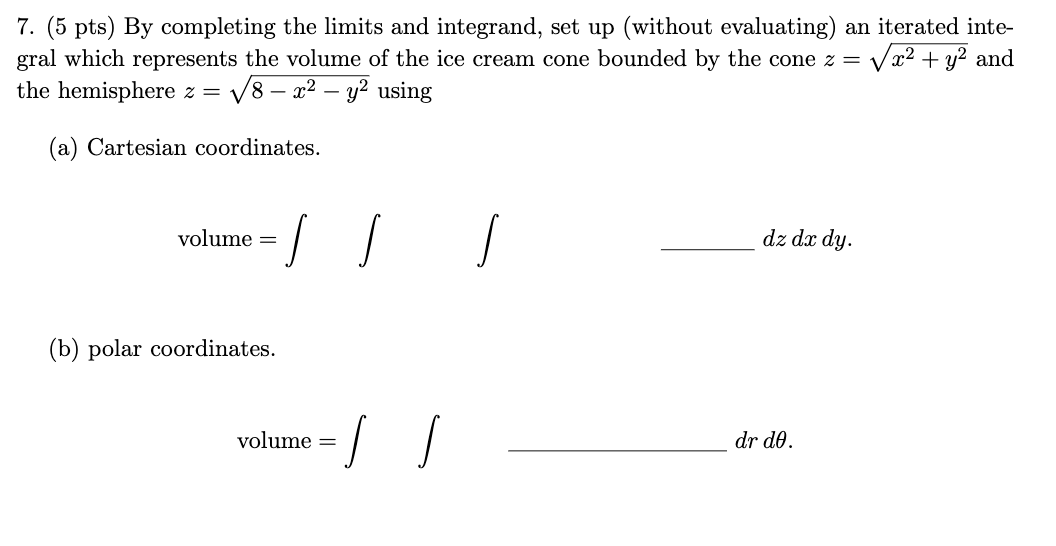7. (5 pts) By completing the limits and integrand, set up (without evaluating) an iterated inte- gral which represents the volume of the ice cream cone bounded by the cone z = V x2 + y2 and the hemisphere z = V8 – x2 - y2 using (a) Cartesian coordinates. volume = dz dx dy. (b) polar coordinates. volume = I dr de.

• ### 7. (5 pts) By completing the limits and integrand, set up (without evaluating) an iterated inte-gral...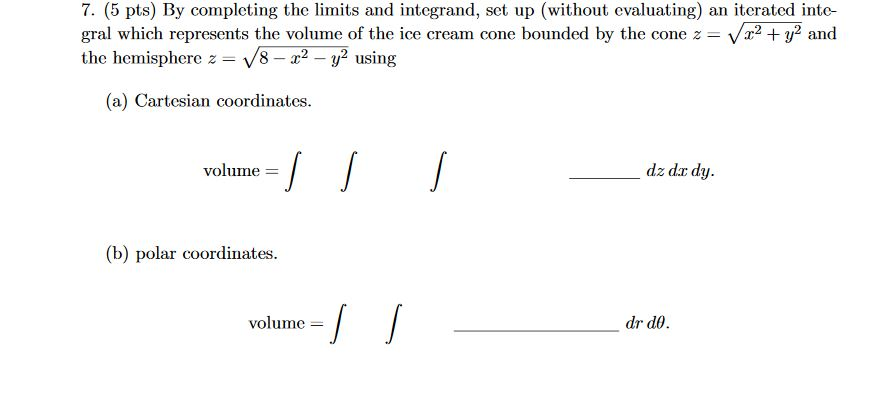7. (5 pts) By completing the limits and integrand, set up (without evaluating) an iterated inte-gral which represents the volume of the ice cream cone bounded by the cone z=√x2+y2andthe hemisphere z=√8−x2−y2using(a) Cartesian coordinates. 7. (5 pts) By completing the limits and integrand, set up (without evaluating) an iterated inte- gral which represents the volume of the ice cream cone bounded by the cone z = Vr2 + y2 and the hemisphere z = 18 - 22 - y2 using...

• ### 6. (4 pts) Consider the double integral∫R(x2+y)dA=∫10∫y−y(x2+y)dxdy+∫√21∫√2−y2−√2−y2(x2+y)dxdy.(a) Sketch the region of integration R in Figure 3.(b)...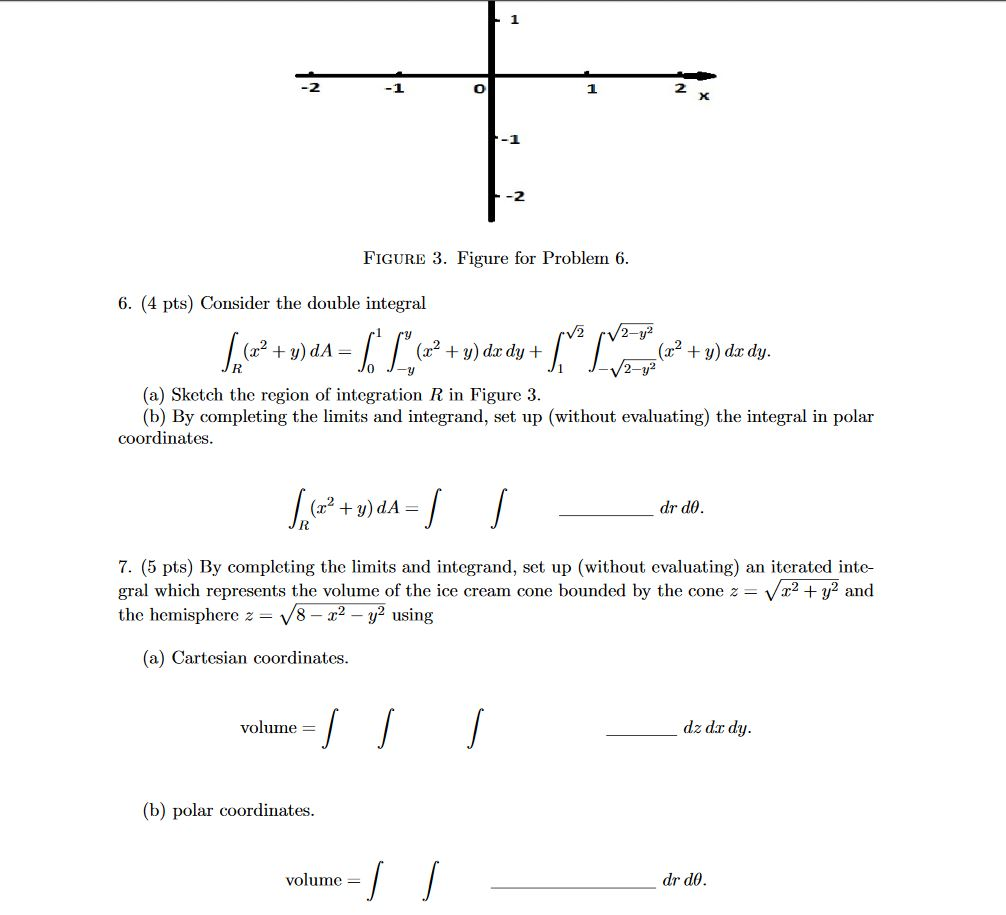6. (4 pts) Consider the double integral∫R(x2+y)dA=∫10∫y−y(x2+y)dxdy+∫√21∫√2−y2−√2−y2(x2+y)dxdy.(a) Sketch the region of integration R in Figure 3.(b) By completing the limits and integrand, set up (without evaluating) the integral in polar coordinates.∫R(x2+y)dA=∫∫drdθ.7. (5 pts) By completing the limits and integrand, set up (without evaluating) an iterated inte-gral which represents the volume of the ice cream cone bounded by the cone z=√x2+y2andthe hemisphere z=√8−x2−y2using(a) Cartesian coordinates.volume =∫∫∫dz dxdy.(b) polar coordinates.volume =∫∫drdθ. -1 -2 FIGURE 3. Figure for Problem 6. 6. (4 pts)...

• ### 6. (4 pts) Consider the double integral∫R(x2+y)dA=∫10∫y−y(x2+y)dxdy+∫√21∫√2−y2−√2−y2(x2+y)dxdy.(a) Sketch the region of integrationRin Figure 3.(b) By completing...6. (4 pts) Consider the double integral∫R(x2+y)dA=∫10∫y−y(x2+y)dxdy+∫√21∫√2−y2−√2−y2(x2+y)dxdy.(a) Sketch the region of integrationRin Figure 3.(b) By completing the limits and integrand, set up (without evaluating) the integral in polar coordinates. -1 -2 FIGURE 3. Figure for Problem 6. 6. (4 pts) Consider the double integral V2 /2-y² + = (x2 + y) dx dy + + y) do dy. 2-y2 (a) Sketch the region of integration R in Figure 3. (b) By completing the limits and integrand, set up (without evaluating)...

• ### Question Use cylindrical coordinates to set up the triple integral needed to find the volume of...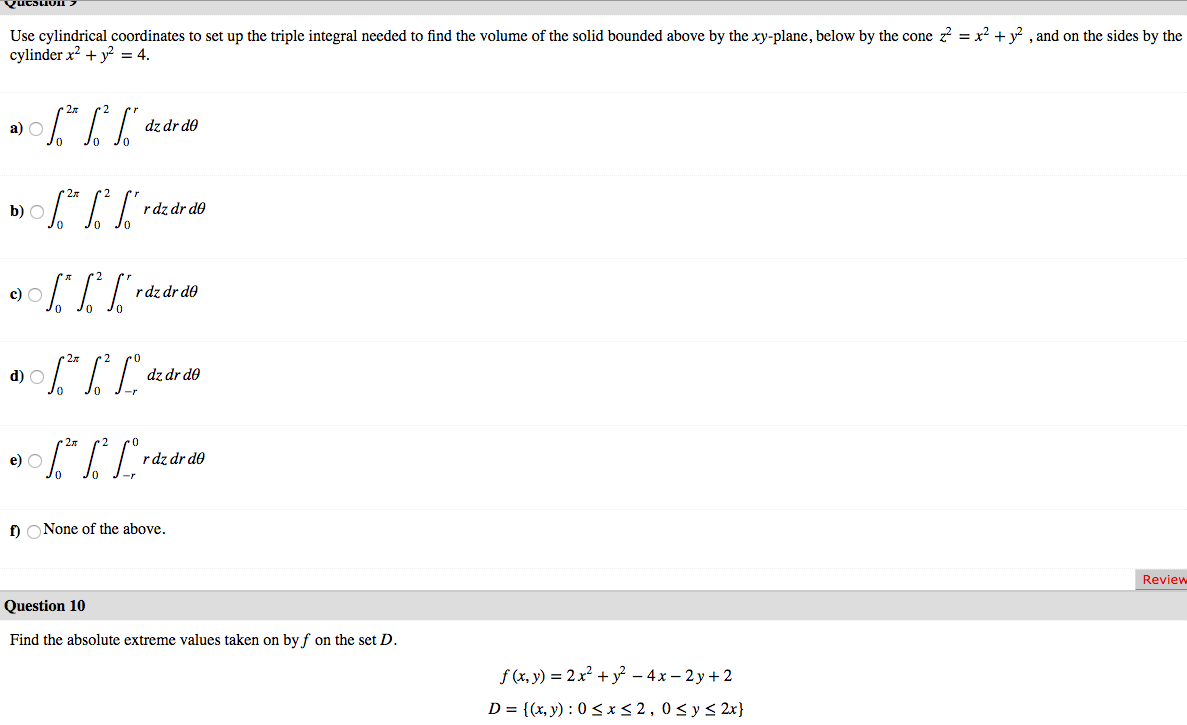Question Use cylindrical coordinates to set up the triple integral needed to find the volume of the solid bounded above by the xy-plane, below by the cone z = x2 + y2 , and on the sides by the cylinder x2 + y2 = 4. a) 06.* %* ["dz dr do b) \$* * S*rde de do JO 0% ] raz dr do a) \$** [Lºdz dr do 0906.*|*Lºrdz dr do 2 po dz dr do Jo J- O J-...

• ### The region is a right circular cone, 2 = Var? + y2 with height 29. Find...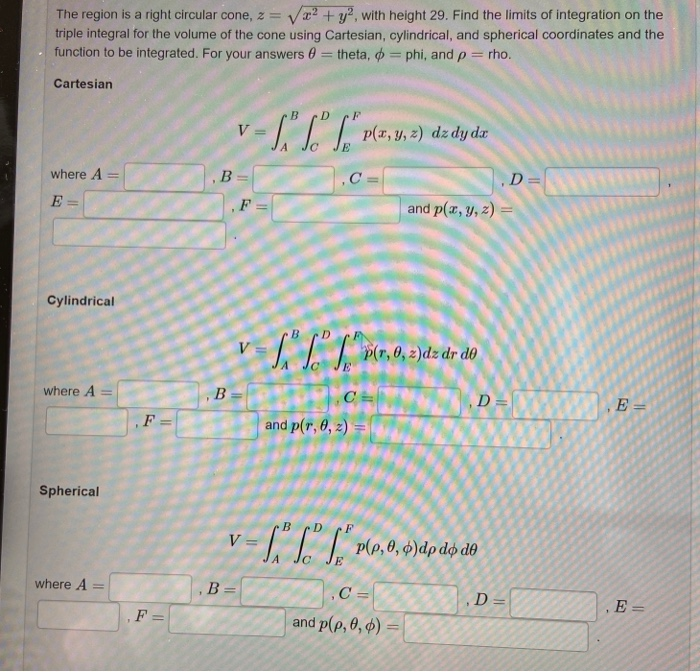The region is a right circular cone, 2 = Var? + y2 with height 29. Find the limits of integration on the triple integral for the volume of the cone using Cartesian, cylindrical, and spherical coordinates and the function to be integrated. For your answers 0 theta, o=phi, and p = rho. Cartesian V = p(x, y, z) dz dy da where A C = B = ,F= E ,D= and p(x, y, z) = Cylindrical V = so" C"S"...

• ### hello this is calculus III class I want to help with these questions to answer it...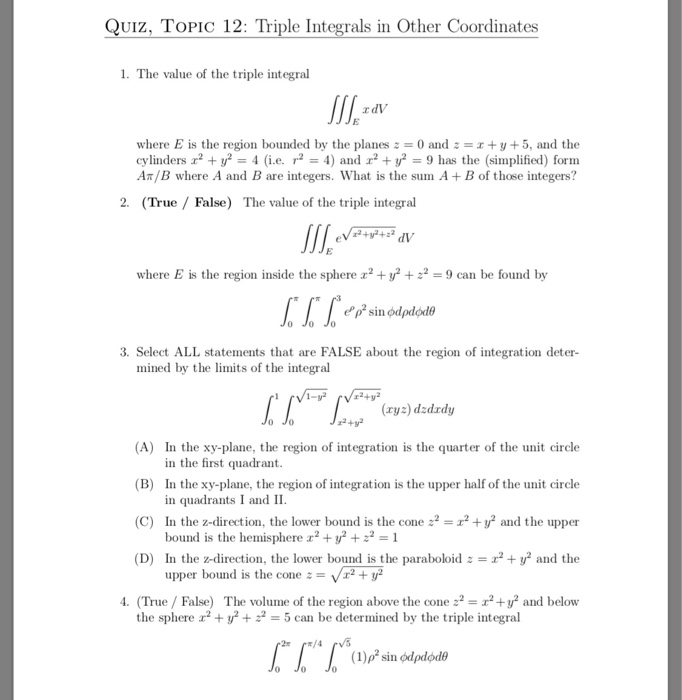hello this is calculus III class I want to help with these questions to answer it Could you help me please with all of them to solve them please thank you QUIZ, TOPIC 12: Triple Integrals in Other Coordinates 1. The value of the triple integral where is the region bounded by the planes 2 = 0 and 2 = 1 + y + 5, and the cylinders r? + y2 = 4 (i.e. r2 = 4) and r2 +...

• ### The region is a cone, z == ? + ytopped by a sphere of radius 4....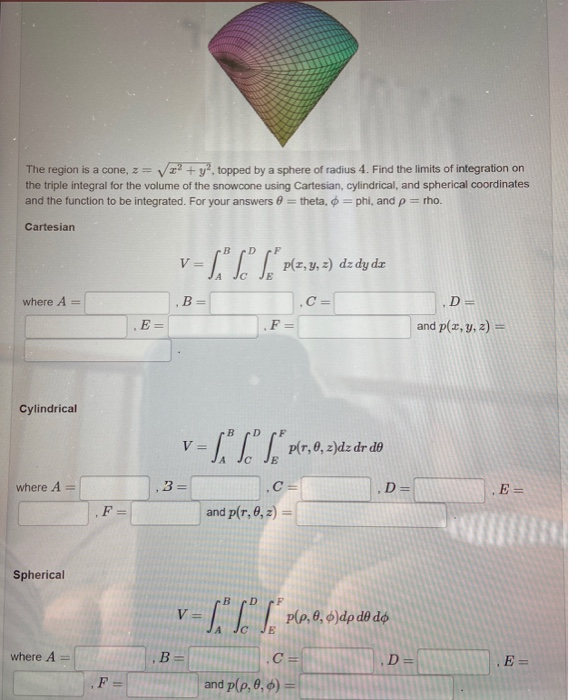The region is a cone, z == ? + ytopped by a sphere of radius 4. Find the limits of integration on the triple integral for the volume of the snowcone using Cartesian, cylindrical, and spherical coordinates and the function to be integrated. For your answers 0 = theta, o =phi, and p = rho. Cartesian V= "SC"}, "plz,y,z2) dz dydz where A B = .D= and p(x, y, z) = E= F= Cylindrical v=L" S "*P10,0,2)dz dr do where...

• ### please show all work in clean and legible handwriting with all labels and steps that is properly explained for PROBLEMS #1, 2, 3, AND 4. Any incorrect answers and not solving all 4 problems...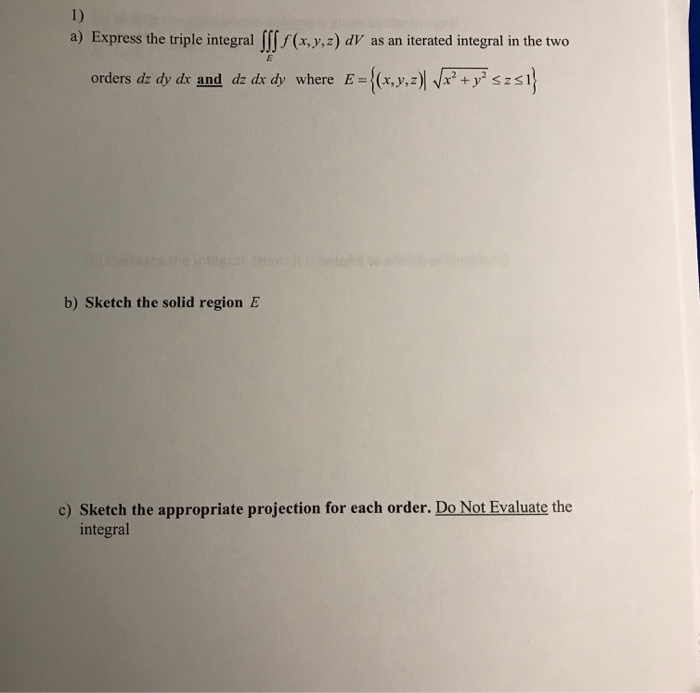please show all work in clean and legible handwriting with all labels and steps that is properly explained for PROBLEMS #1, 2, 3, AND 4. Any incorrect answers and not solving all 4 problems will get an immediate thumbs down because they did not follow directions, thank you 1) Express the triple integral Ⅲf (x,y,z) dV as an iterated integral in the two a) E={(x,y,z)Wr2+yszaj orders dzdy dr and dz dr dy where b) Sketch the solid region E c)...